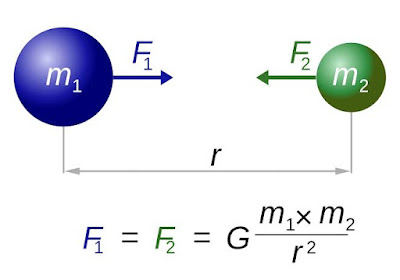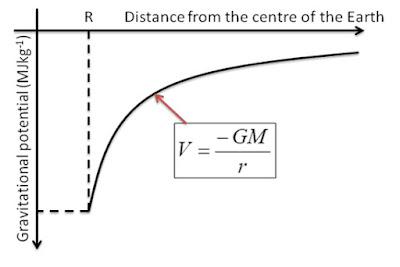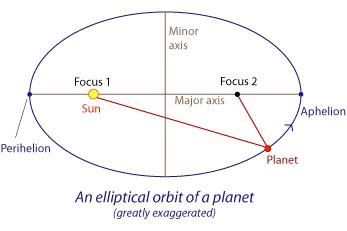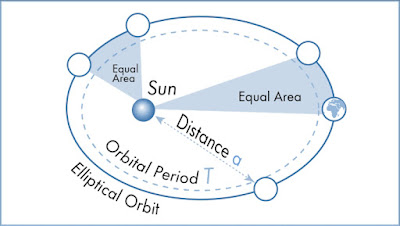# GRAVITATION study notes for IIT JEE | concept booster , chapter highlights.

SHARING INCREASES KNOWLEDGE

# GRAVITATION

## NEWTON’S LAWS OF GRAVITATION

This laws states that every body in the universe attract another body with a force which is directly proportional to product of the their masses and inversely proportional to the square of distance between them act along the line joining the two bodies.
According to this law-

F = GMm/r^2 , where G is the proportionality constant called universal Gravitational constant.1. This universal Gravitational constant G is a scaler quantity.
2.  This constant remains same or unchanged in this entire universe , and it is independent of the shape , size and nature of the object and also independent of the intervening medium and temperature of the bodies.
3. Value of G is in SI system is 6.67×10^-11Nm^2/kg^2. and value of G in CGS system is 6.67×10^-8 dye.cm^2/g^2
4. Dimensional formula of G is[M^-1.L^3T^-2]
5. Gravitational force between the two bodies is equal in magnitude but in opposite directions , hence it obeys the Newton’s third law of motion. It forms action reaction pair.
6. This is a type of conservative force , means work done by it is independent of path taken.
7. It is central force because it acts along the line joining the centres of the two bodies.

## ACCELERATION DUE TO GRAVITY

Acceleration produced in the body due to Gravitational force is called acceleration due to gravity, Or in another word, It is the acceleration produced in the body which is falling free in the effect of gravity.
Acceleration due to gravity is denoted by small letter g and it’s unit is m/s^2.
Mathematically it is given as-
g = GMe/r^2.
where Me is the mass of the earth and r is the distance of the body from the centre of the earth.
1. If the body is on the surface of the earth then g is given as, g = GMe/Re^2.    Where Me is the earth’s mass and r=Re is equal to radius of the earth.
2. It is a vector quantity and it’s dimensional formula is [M^0L^1T^-2]
3. Value of g on the surface of the earth is taken as 9.8m/s^2.
4. It’s value can change with change of the altitude ,depth, shape and rotation of the body.
5. Gravitational acceleration in the form of Gravitational constant is -.                            g = (4/3)πGReρ , where Re is the radius of the earth and ρ is the density of the earth.
6. The value of acceleration due to gravity is independent of the shape, size and mass of the body, which is experiencing the Gravitational force. But it depends on the mass and radius of earth or any other planets which exerts Gravitational force.
7. Acceleration due to gravity increased/decreased by 2n% on the surface of the planets, if their radius is decreased/increased by n% ,if their mass keeps unchanged.
8. Acceleration due to gravity increased by n% on the surface of the planets, if their mass is increased by n% ,if their radius keeps unchanged.
9. Acceleration due to gravity decreased by n% if the density of the planets decreased by n%, keeping the radius unchanged.

### VARIATIONS IN ACCELERATION DUE TO GRAVITY

#### DUE TO ALTITUDE (h)

If we go high through the height h from the earth crust then the acceleration due to gravity starts decreasing.
At height h from the earth crust ADG is given as-
g(h) = GMe/(Re+h)^2 = g(1+h/Re)^-2
If h<<<<Re then
g(h) = g(1-2h/Re).   [g = GMe/Re^2]

#### DUE TO DEPTH (d)

When we go below towards the centre of the earth , acceleration due to gravity starts decreasing and it’s become zero at centre.
It’s again starts increasing if we starts to go towards the crust and it become maximum at the surface of the earth. So acceleration due to gravity at depth d is given as-
g(d) = GMe(Re-d)/Re^3 = g(1-d/Re)

#### DUE TO ROTATION OF THE EARTH

If earth is rotating with angular velocity ω , then the acceleration due to gravity because of rotating of the earth at any latitude δ is given as-
g(δ) = g-Reω^2.cos^2δ
The angular velocity(ω) of the earth is taken 7.3×10^-5rad/s.
1. At the equator where δ is 0 , the  g(δ) is given as ,   g(δ)  = g – ω^2.Re
2. At the poles , δ is 90° , the g(δ) is given as , g(δ) = g
3. Difference between the acceleration due to gravity at poles and equator is -.   g(p)-g(e) = g-(g-Reω^2) = Reω^2

#### DUE TO THE SHAPE OF THE EARTH

Earth is not a perfectly sphere , it is flattened at the poles and bulged at the equator as same like as an orange, due to this flatting the diameter of the earth is 21 km larger along with the equator as compared to diameter along with the poles. Both rotation and equatorial bulged contribute additively keeps the g smaller at the equator than the poles.

## GRAVITATIONAL FIELD1. Gravitational field is a space around a body in which if another body comes then it experiences a Gravitational pull.
2. The Gravitational field intensity (E) at a distance r due to a point mass M is -.           E = GM/r^2 = Gravitational acceleration
3. Gravitational field intensity is a vector quantity it always directed towards the centre of the mass which produces Gravitational the field.
4. E become zero at infinity.
5. Gravitational field intensity obeys the superposition principle ie. the total G-intensity(E) at a point is the vector sum of all the G-intensities acting at that point due to individual masses. ,                                    E = E1+E2+E3+……
6. Gravitational field intensity due to a point mass is E = GM/r^2
7. Gravitational field intensity due to uniform circular ring along it axis is ,.                        E = GMr/(r^2+R^2)^3/2 , where R is the radius of the ring and r is distance of the point from its centre.
8. Gravitational field intensity due to a uniform disk at a point on its axis is.            E = (2GMr/R^2)[1/r – 1/√(r^2+R^2)].          In terms of θ is.                                      E = 2GM(1-cosθ)/R^2.           where R is the radius of the ring and r is distance of the point from its centre.
9. Gravitational field intensity due to a solid sphere of radius R at any external point of distance r from its centre.                             E = GM/r^2 , and any internal point of distance r , E = GMr/R^3
10. Gravitational field intensity due to uniform hollow sphere at any point inside the sphere is zero. And at any point outside the sphere of distance r is. ,E = GM/r^2

## GRAVITATIONAL POTENTIAL

It is the amount of work done in bringing a body of unit mass from infinite to a point in Gravitational field of another body without any acceleration.
Mathematically ;

V = W/m1. Gravitational potential at a point due to a point mass M is , V = -GM/r
2. Gravitational potential due to uniform ring at a point along its axis is ,                                   V = -GM/√(R^2+r^2)
3. Gravitational potential due to hollow sphere , outside the shell is V = -GM/r.     And inside the shell is V = -GM/R.
4. Gravitational potential V is a scalar quantity but potential gradient dV/dr is a vector quantity.
5. The relation between Gravitational potential and Gravitational intensity is.       E =-dV/Dr
6. Gravitational potential obeys the superposition principle ie. The sum of all the Gravitational potential V at a point is sum of all the individual Gravitational potential which are acting at that point.                                      V = V1 +V2+V3+……
7. Gravitational potential due to a uniform solid sphere of radius R , when the point is outside the sphere is.           V = -GM/r. and when the point is inside the sphere is.                                                V = -GM(3R^2 – r^2)/2R^3

## GRAVITATIONAL POTENTIAL ENERGY

Gravitational potential energy is the amount of work done in bringing a body of unit mass in the Gravitational field of other body from infinite without any acceleration.
Mathematically
W = Vm
1. Gravitational potential energy of a body of mass m which is r distance from the centre of mass M is, U = -GMm/r
2. Work done in taking a body of mass m from the earth’s surface to the height h , against the Gravitational pull is-.              W = ∆U = GMmh/R(R+h) , if h<<<R then the W = GMmh/R^2 = mgh , if h=R then the W = mgh/2

## KEPLER’S LAWS OF PLANETARY MOTION

#### LAW’S OF ORBITThis laws states that, all planets moves in elliptical orbit with the sun situated at one of the foci of the ellipse.
This laws gives the geometry of planetary motion.

#### LAW’S OF AREAThis laws states that , the speed of planets around the sun varies in such a way that the radius vector drawn from the sun to the planets sweep equal areas in equal interval of time.

#### LAW’S OF PERIOD

This laws states that, the square of the time period of revolution of the planets around the sun is directly proportional to the cube of the semi major axis of the elliptical orbit.

## ESCAPE VELOCITY

It is the minimum speed with which a body is projected vertically upward from the surface of the earth , that it escaped out from the Gravitational pull of the earth and never will return.
Mathematically
Ve = √(2GM/R) , where M is the mass of the planet and R is the radius of that planet.
Ve = √(2G×volume×density/R)
= √[(2G/R)(4/3πR^3)ρ] =√[8πGR^2ρ/3]
1. Escape velocity for earth is 11.2km/sec
2. To throw a heavy or light object out of the earth you need same velocity.
3. If the ratio of radii of two planets is r and the ratio of their acceleration due to gravity is a , then the ratio their escape velocity is   √ar.
4. Escape velocity in the terms of g is-.             Ve = √2gr
5. If radius of the earth is doubled keeping mass unchanged then the escape velocity become 1/√2 times the present value.

## SATELLITE

Satellite is a body , which is constantly revolving around a body(which is greater in size) in the fixed orbit.
If a satellite of mass m revolving around the planet of mass M at the height h from the surface, then the radius of the orbit is,                 r = R+h
Where R is the radius of the planet. The Gravitational force between m and M provide the necessary centripetal force for circular motion.

#### ORBITAL VELOCITY

At which speed satellites revolve around the planets is called orbital velocity.
1. Orbital speed is independent of the mass of the orbiting body and it is always directed along the tangent of the orbit.
2. Orbital speed is given by Vo = √GM/(R+h). if h= 0 , means satellite is very close to the planet(earth) , then Vo = √GM/R = √gR                     = √10×6.4×10^6 = 8km/sec
3. Orbital velocity in the terms of escape velocity is Vo = √2GM/2R = Ve/√2 Or  Ve = √2.Vo
4. Orbital velocity vary near the surface of the earth and it is about 7.92Km/s
5. If satellite altitude is n times the radius of the earth, then the orbital velocity is.    1/√(1+n) ,times orbital velocity near the earth surface.
6. If satellite radius is n times the radius of the earth, then the orbital velocity will be     1/√n ,times the orbital velocity near the earth surface.

#### TIME PERIOD OF THE SATELLITE

It is the time taken by the satellites to complete one revolution. It is denoted by T , it is given by
T = 2πr/v = 2πr(√r/GM)
After squaring both sides
T^2 = (4π^2/GM)r^3,
[ T^2 directly proportional to r^3 , Kepler’s laws of period]
The value of proportionality constant is
(4π^2/GM)

#### ANGULAR MOMENTUM OF THE SATELLITE

Angular momentum is given as
L= mvr = mr√GM/r = √m^2.GMr
In the satellites motion the net force is centripetal force , and torque due to this force is zero about the centre of the orbit. So total angular momentum is conserved in this case.
L is constant.

#### ENERGY OF THE SATELLITE

The potential energy of the satellite is –
U = mV = – GMm/r , the kinetic energy of the satellite is K.E = 1/2mVo^2 = GMm/2r
Total mechanical energy of the satellite is
Total(energy) = – GMm/r +GMm/2r = -GMm/2r
The total energy is negative means satellite is bound to the planet with a attractive force and external energy is necessary to remove the satellite from the orbit to infinity.

#### BINDING ENERGY OF THE SATELLITE

It is the energy required to remove the satellite from the orbit to the infinity.
Binding energy, E = -GMm/2r

#### GEOSTATIONARY SATELLITE

It is the type of satellites , which rotates in the same direction as earth rotates ie. West to east
Then the relative angular velocity of both bodies is zero. , ωs – ωe = 0.
So for a observer who is on the earth , satellite appears stationary, such satellite called geo-stationary satellite. The orbit of geo-stationary satellite is called parked orbit.
1. Time period is T= 24 hours.
2. Height of geo-stationary satellite is given as h = [T^2.GMe/4π^2]^1/3 – Re = 36,000km
3. This types of satellites are used in radio communication, transmission of TV and telephone signals.

#### POLAR SATELLITE

The satellites which revolve around the earth in circular motion from north to south orbit and passes over the north to south pole.
1. It is low altitude satellite ~500-800km
2. Angle of inclination from equatorial plane is 90°.
3. They often are called sun synchronous satellite because it croses the earth equator daily at the same time.
4. These satellites are used in remote sensing and meteorological research and enviromental studies.etc.

### WEIGHTLESSNESS IN SATELLITE

The radial acceleration of the satellite is given by ar = Fr/m = GMm/r^2.m = GM/r^2
For a astronaut in the satellite , his normal reaction N and his radial force m(a).a(r) balances the Gravitational force as follows
GMm/r^2 – N – m(a).a(r) = 0, or
GMm/r^2 – N – GMm(a)/r^2 = 0 , then N = 0
So a astronaut feel no gravity in the satellite.
SHARING INCREASES KNOWLEDGE